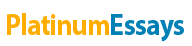# Analysis of Chotukool Market[

By:   •  August 5, 2019  •  Case Study  •  1,286 Words (6 Pages)  •  2,550 Views

Page 1 of 6

Analysis of Chotukool Market[pic 1][pic 2]

An assignment for
Managerial Statistics (ANT 5001)

by

FAS: L1

ANKITA BAJPAI – 19F208

ARJUN MALLYA – 19F211

KRISHNA SHARMA – 19F222

SHUBHAM JOSHI – 19F254

Under the Guidance of

Prof. Ganes M. Pandya

[pic 3]

T.A. PAI MANAGEMENT INSTITUTE

MANIPAL, INDIA

August 2019

Motivation

Godrej has introduced Chotukool – A compact refrigerator. For every product to be a success, customer needs must be well understood and analysed. Thus, we conducted a survey of three regions where we want to establish our business – urban, semi-urban and rural. An experiment was conducted in this case to find out the success of Chotukool in all the 3 regions.

URBAN REGION – Availability of electricity is 24 hrs

SEMI URBAN REGION – Availability of electricity is 16 hrs

RURAL REGION – Availability of electricity is 12 hrs

We took a sample of total 300 people for our survey to find out the success of Chotukool in each region. The data collected is present in Contingency Table

1. We conducted a survey in 3 regions, with a sample size of 300 each. What is the probability of acceptance of Chotukool in urban area?
2. What is the cross-regional likeability of Chotukool?
1. Probability of Chotukool liked given that it belongs to region.
2. Given that chotukool is already liked, what is the probability it from rural region?

METHOD:

For solving first question we have used the concepts of Simple Probability. For solving second question we have used Marginal and Joint Probability. For third question we have used Conditional probability. We have created a contingency table for better data analysis. The results from one question is used as an input to the subsequent questions. For solving Fourth question we have used Bayes Theorem to get the result.

Contingency Table:

 Row Labels Liked Not Liked Grand Total Rural 69 31 100 Semi-Urban 45 55 100 Urban 45 55 100 Grand Total 159 141 300

Events:

 Experiment Event Name Chotukool is liked in Rural Region A1 Chotukool is liked in Urban Region A2 Chotukool is liked in Semi-Urban Region A3 Out of total those who liked ChotuKool, how many belongs to Semi Urban Region A4 Out of total those who liked ChotuKool, how many belongs to Urban Region A5 ChotuKool Being liked in both Semi Urban AND Urban Region A6 ChotuKool Being liked in both Semi Urban OR Urban Region A7 Chotukool liked given that it belongs to Urban region A8 Chotukool liked given that it belongs to Semi Urban region A9 Chotukool liked given that it belongs to Rural region A10

Probabilities

1. Simple Probability – Defined as “The probability of occurrence of a simple event”

In the given case –

1. Probability of Chotukool being liked in the Rural region=

(Number of People liked Chotukool in Rural Region)/(Total No. of people in Rural Region)

i.e P(A1) = 69 / 100 = 0.69

1. Probability of Chotukool being liked in the Semi Urban region=

(Number of People liked Chotukool in Semi Urban Region)/(Total No. of people in Semi Urban Region)

i.e P(A2) = 45 / 100 = 0.45

1. Probability of Chotukool being liked in the Urban region=

(Number of People liked Chotukool in Urban Region)/(Total No. of people in Urban Region)

i.e P(A3) = 45 / 100 = 0.45

1. Probability of “Out of total those who liked ChotuKool, how many belongs to Semi Urban Region” = (Number of People liked Chotukool belonging from Semi Urban Region)/(Total number of people who liked Chotukool)

i.e P(A4) = 45 / 159

P(A4) = 0.283

1. Probability of “Out of total those who liked ChotuKool, how many belongs to Urban Region” = (Number of People liked Chotukool belonging from Urban Region)/(Total number of people who liked Chotukool)

i.e P(A5) = 45 / 159

P(A5) = 0.283

1. Marginal and Joint Probability – Defined as “The probability of Two independent events happening together”

In this case –

1. Probability of ChotuKool Being liked in both Semi Urban AND Urban Region =

Probability of Chotukool being liked in the Semi Urban region * Probability of Chotukool being liked in the Urban region.

P(A6) = P(A2) * P(A3) = 0.45*0.45

P(A6) = 0.2025

1. Probability of ChotuKool Being liked in both Semi Urban OR Urban Region =

Probability of “Out of total those who liked ChotuKool, how many belongs to Semi Urban Region” + Probability of “Out of total those who liked ChotuKool, how many belongs to Urban Region”.

P(A7) = P(A5) + P(A6) = 0.283 + 0.283

...